# Time Series Analysis: The Process of Seasonal Adjustment

WHAT ARE THE TWO MAIN PHILOSOPHIES OF SEASONAL ADJUSTMENT?

The two main philosophies for seasonal adjustment are the model based method and the filter based method.

Filter based methods

This method applies a set of fixed filters (moving averages) to decompose the time series into a trend, seasonal and irregular component.

The underlying notion is that economic data is made up of a range of cycles, including business cycles (the trend), seasonal cycles (seasonality) and noise (the irregular component). A filter essentially removes or reduces the strength of certain cycles from the input data.

To produce a seasonally adjusted series from data collected monthly, events that occur every 12, 6, 4, 3, 2.4 and 2 months need to be removed. These correspond to seasonal frequencies of 1, 2, 3, 4, 5 and 6 cycles per year. The longer non-seasonal cycles are considered to be part of the trend and the shorter non-seasonal cycles form the irregular. However the boundary between the trend and irregular cycles can vary with the length of the filter used to obtain the trend. In ABS seasonal adjustment, cycles which contribute significantly to the trend are typically larger than about 8 months for monthly series and 4 quarters for quarterly series.

The trend, seasonal and irregular components do not need explicit individual models. The irregular component is defined as what remains after the trend and seasonal components have been removed by filters. Irregulars do not display white noise characteristics.

Filter based methods are often known as X11 style methods. These include X11 (developed by U.S Census Bureau), X11ARIMA (developed by Statistics Canada), X12ARIMA (developed by U.S Census Bureau), STL, SABL and SEASABS (the package used by the ABS)..

Computational differences between various methods in X11 family are chiefly the result of different techniques used at the ends of the time series. For example, some methods use asymmetric filters at the ends, while other methods extrapolate the time series and apply symmetric filters to the extended series.

Model based methods

This approach requires the trend, seasonal and irregular components of the time series to be modelled separately. It assumes the irregular component is “white noise” - that is all cycle lengths are equally represented. The irregulars have zero mean and a constant variance. The seasonal component has its own noise element.

Two widely used software packages which apply model based methods are STAMP and SEATS/TRAMO (developed by the Bank of Spain).

Major computational differences between the various model based methods are usually due to model specifications. In some cases, the components are modelled directly. Other methods require the original time series to be modelled first, and the component models decomposed from that.

WHAT IS A FILTER?

Filters can be used to decompose a time series into a trend, seasonal and irregular component. Moving averages are a type of filter that successively average a shifting time span of data in order to produce a smoothed estimate of a time series. This smoothed series can be considered to have been derived by running an input series through a process which filters out certain cycles. Consequently, a moving average is often referred to as a filter.

The basic process involves defining a set of weights of length m1 + m2 + 1 as: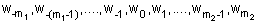Note: a symmetric set of weights has m1=m2 and wj = w-j

A filtered value at time t can be calculated by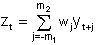where Yt describes the value of the time series at time t.

For example, consider the following series: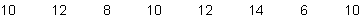Using a simple 3 term symmetric filter (i.e. m1= m2= 1 and all weights are 1/3), the first term of the smoothed series is obtained by applying the weights to the first three terms of the original series: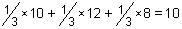The second smoothed value is produced by applying the weights to the second, third and fourth terms in the original series: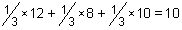And so on…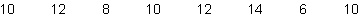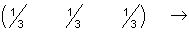The smoothed series becomes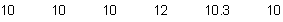WHAT IS THE END POINT PROBLEM?

Reconsider the series: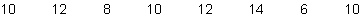This series contains 8 terms. However, the smoothed series obtained by applying symmetric filter to the original data contains only 6 terms:This is because there is insufficient data at the ends of the series to apply a symmetric filter. The first term of the smoothed series is a weighted average of three terms, centered on the second term of the original series. A weighted average centered on the first term of the original series cannot be obtained as data before this point is not available. Similarly, it is not possible to calculate a weighted average centered on the last term of the series, as there is no data after this point.

For this reason, symmetric filters cannot be used at either end of a series. This is known as the end point problem. Time series analysts can use asymmetric filters to produce smoothed estimates in these regions. In this case, the smoothed value is calculated ‘off centre’, with the average being determined using more data from one side of the point than the other according to what is available. Alternatively, modelling techniques may be used to extrapolate the time series and then apply symmetric filters to the extended series.

HOW DO WE DECIDE WHICH FILTER TO USE?

The time series analyst chooses an appropriate filter based on its properties, such as which cycles the filter removes when applied. The properties of a filter can be investigated using a gain function.

WHAT IS A GAIN FUNCTION?

Gain functions are used to examine the effect of a filter at a given frequency on the amplitude of a cycle for a particular time series. The following diagram is the gain function for the symmetric 3 term filter we studied earlier:

Figure 1: Gain Function for Symmetric 3 Term Filter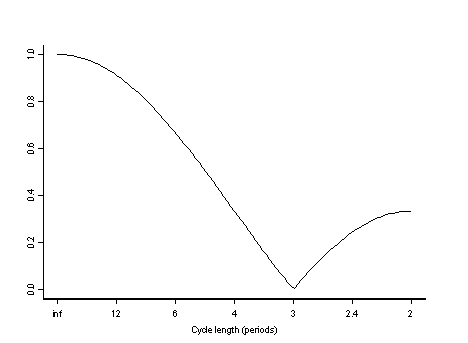The horizontal axis represents the length of an input cycle relative to the period between observation points in the original time series. So an input cycle of length 2 is completed in 2 periods, which represents 2 months for a monthly series, and 2 quarters for a quarterly series. The vertical axis shows the amplitude of the output cycle relative to an input cycle.

This filter reduces the strength of 3 period cycles to zero. That is, it completely removes cycles of approximately this length. This means that for a time series where data is collected monthly, any seasonal effects which occur quarterly will be eliminated by applying this filter to the original series.

WHAT IS A PHASE SHIFT?

A phase shift is the time shift between the filtered cycle and the unfiltered cycle. A positive phase shift means that the filtered cycle is shifted backwards and a negative phase shift it is shifted forwards in time.

Phase shifting occurs when timing of turning points is distorted, for example when the moving average is placed off-centre by the asymmetric filters. That is they will occur either earlier or later in the filtered series, than in the original. Odd length symmetric moving averages (as used by the ABS), where the result is centrally placed, do not cause time phase shifting. It is important for filters used to derive the trend to retain the time phase, and hence the timing of any turning points.

Figures 2 and 3 show the effects of applying a 2x12 symmetric moving average which is off-centre. The continuous curves represent the initial cycles and the broken curves represents the output cycles after applying the moving average filter.

 Figure 2: 24 Month Cycle, Phase = -5.5 months Amplitude = 63%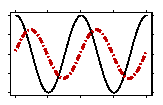Figure 3: 8 Month Cycle, Phase = -1.5 months Amplitude = 22%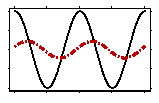WHAT ARE HENDERSON MOVING AVERAGES?

Henderson moving averages are filters which were derived by Robert Henderson in 1916 for use in actuarial applications. They are trend filters, commonly used in time series analysis to smooth seasonally adjusted estimates in order to generate a trend estimate. They are used in preference to simpler moving averages because they can reproduce polynomials of up to degree 3, thereby capturing trend turning points.

The ABS uses Henderson moving averages to produce trend estimates from a seasonally adjusted series. The trend estimates published by the ABS are typically derived using a 13 term Henderson filter for monthly series, and a 7 term Henderson filter for quarterly series.

Henderson filters can be either symmetric or asymmetric. Symmetric moving averages can be applied at points which are sufficiently far away from the ends of a time series. In this case, the smoothed value for a given point in the time series is calculated from an equal number of values on either side of the data point.

To obtain the weights, a compromise is struck between the two characteristics generally expected of a trend series. These are that the trend should be able to represent a wide range of curvatures and that it should also be as smooth as possible.

The weighting patterns for a range of symmetric Henderson moving averages are given in the following table:

 Filter Length Symmetric Weighting Pattern for Henderson Moving Average 5 Term (-0.073, 0.294, 0.558, 0.294, -0.073) 7 Term (-0.059, 0.059, 0.294, 0.412, 0.294, 0.059, -0.059) 9 Term (-0.041, -0.010, 0.119, 0.267, 0.330, 0.267, 0.119, -0.010, -0.041) 13 Term (-0.019, -0.028, 0.0, 0.066, 0.147, 0.214, 0.240, 0.214, 0.147, 0.066, 0.0, -0.028, -0.019) 23 Term (-0.004, -0.011, -0.016, -0.015, -0.005, 0.013, 0.039, 0.068, 0.097, 0.122, 0.138, 0.148, 0.138, 0.122, 0.097, 0.068, 0.039, 0.013, -0.005, -0.015, -0.016, -0.011, -0.004)

The corresponding gain functions, which represent the strength remaining at various cycle lengths after the filter is applied, are below:

 Figure 4: 5 Term Symmetric Henderson Trend Filter Figure 5: 7 Term Symmetric Henderson Trend Filter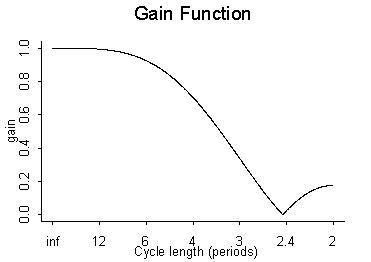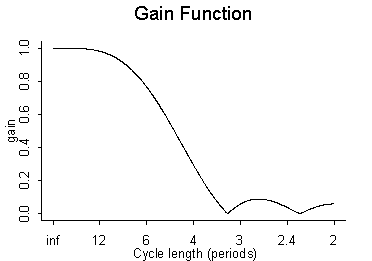Figure 6: 9 Term Symmetric Henderson Trend Filter Figure 7: 13 Term Symmetric Henderson Trend Filter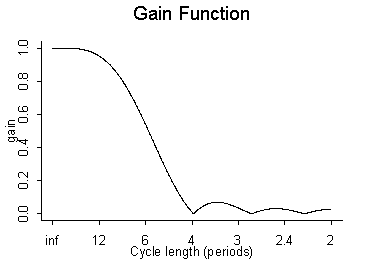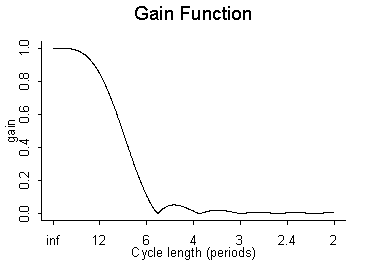Figure 8: 23 Term Symmetric Henderson Trend Filter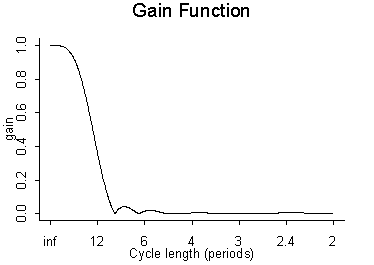In general, the longer the trend filter, the smoother the resulting trend, as is evident from a comparison of the gain functions above. A 5 term Henderson reduces cycles of about 2.4 periods or less by at least 80%, while a 23 term Henderson reduces cycles of about 8 periods or less by at least 90%. In fact a 23 term Henderson filter completely removes cycles of less than 4 periods.

Henderson moving averages also dampen the seasonal cycles to varying degrees. However the gain functions in Figures 4-8 show that annual cycles in monthly and quarterly series are not dampened significantly enough to justify applying a Henderson filter directly to original estimates. This is why they are only applied to a seasonally adjusted series, where the calendar related effects have already been removed with specifically designed filters.

Figure 9 shows the smoothing effects of applying a Henderson filter to a series:
Figure 9: 23-Term Henderson Filter - Value of Non-residential Building Approvals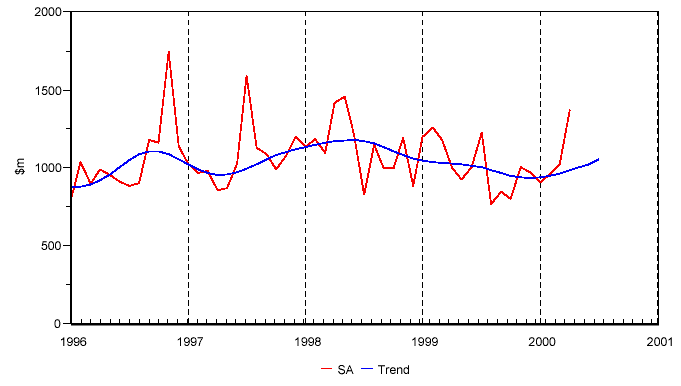HOW DO WE DEAL WITH THE END POINT PROBLEM?

The symmetric Henderson filter can only be applied to regions of data that are sufficiently far away from the ends of the series. For example the standard 13 term Henderson can only be applied to monthly data that is at least 6 observations from the start or end of the data. This is because the filter smoothness the series by taking a weighted average of the 6 terms on either side of the data point as well as the point itself. If we attempt to apply it to a point that is less than 6 observations from the end of the data, then there is not enough data available on one side of the point to calculate the average.

To provide trend estimates of these data points, a modified or asymmetric moving average is used. Calculation of asymmetric Henderson filters can be generated by a number of different methods which produce similar, but not identical results. The four main methods are the Musgrave method, the Minimisation of the Mean Square Revision method, the Best Linear Unbiased Estimates (BLUE) method, and the Kenny and Durbin method. Shiskin et. al (1967) derived the original asymmetric weights for the Henderson moving average which are used within the X11 packages.

Consider a time series where the last observed data point occurs at time N. Then a 13 term symmetric Henderson filter cannot be applied to data points which are measured at any time after and including time N-5. For all these points, an asymmetric set of weights must be used. The following table gives the asymmetric weighting pattern for a standard 13 term Henderson moving average.

 WeightsDatafor period N-12 N-11 N-10 N-9 N-8 N-7 N-6 N-5 N-4 N-3 N-2 N-1 N N 0 0 0 0 0 0 -0.092 -0.058 0.012 0.12 0.244 0.353 0.421 N-1 0 0 0 0 0 -0.043 -0.038 0.002 0.08 0.174 0.254 0.292 0.279 N-2 0 0 0 0 -0.016 -0.025 0.003 0.068 0.149 0.216 0.241 0.216 0.148 N-3 0 0 0 -0.009 -0.022 0.004 0.066 0.145 0.208 0.23 0.201 0.131 0.046 N-4 0 0 -0.011 -0.022 0.003 0.067 0.145 0.21 0.235 0.205 0.136 0.05 -0.018 N-5 0 -0.017 -0.025 0.001 0.066 0.147 0.213 0.238 0.212 0.144 0.061 -0.006 -0.034 N-6 -0.019 -0.028 0 0.066 0.147 0.214 0.24 0.214 0.147 0.066 0 -0.028 -0.019

The asymmetric 13 term Henderson filters do not remove or dampen the same cycles as the symmetric 13 term Henderson filter. In fact the asymmetric weighting pattern used to estimate the trend at the last observation amplifies the strength of 12 period cycles. Also asymmetric filters produce some time phase shifting.

WHAT ARE SEASONAL MOVING AVERAGES?

Almost all of the data investigated by the ABS have seasonal characteristics. Since the Henderson moving averages used to estimate the trend series do not eliminate seasonality, the data must be seasonally adjusted first using seasonal filters.

A seasonal filter has weights which are applied to same period over time. An example of the weighting pattern for a seasonal filter would be:

(1/3, 0, 0, 0, 0, 0, 0, 0, 0, 0, 0, 0, 1/3, 0, 0, 0, 0, 0, 0, 0, 0, 0, 0, 0, 1/3)

where, for instance, a weight of one third is applied to three consecutive Januarys.

Within X11, a range of seasonal filters are available to choose from. These are a weighted 3-term moving average (ma) S3x1, weighted 5-term ma S3x3, weighted 7-term ma S3x5, and a weighted 11-term ma S3x9.

The weighting structure of weighted moving averages of the form, Snxm, is that a simple average of m terms calculated, and then a moving average of n of these averages is determined. This means that n+m-1 terms are used to calculate each final smoothed value.

For example, to calculate an 11-term S3x9, a weight of 1/9 is applied to the same period in 9 consecutive years. Then a simple 3 term moving average is applied across the averaged values: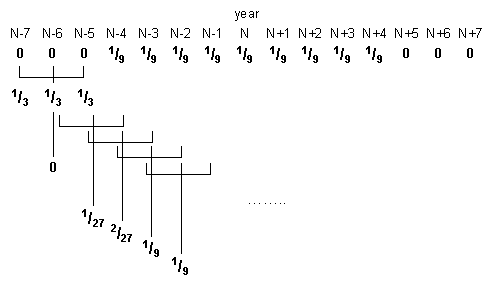This gives a final weighting pattern of (1/27, 2/27, 1/9, 1/9, 1/9, 1/9, 1/9, 1/9, 1/9, 2/27, 1/27).

The gain function for an 11 term seasonal filter, S3x9, looks like:

Figure 10: Gain Function for 11 Term (S3x9) Seasonal Filter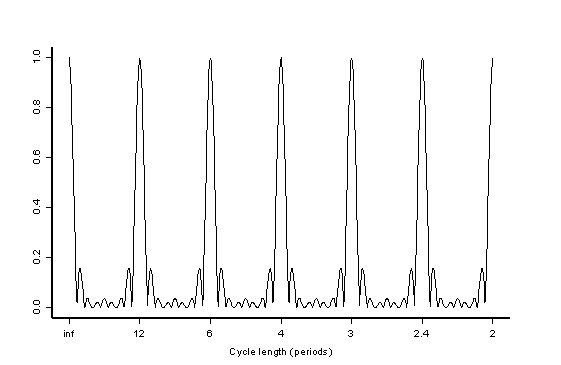Applying a seasonal filter to data will generate an estimate of the seasonal component of the time series, as it preserves the strength of seasonal harmonics and dampens cycles of non-seasonal lengths.

Asymmetric seasonal filters are used at the ends of the series.

WHY ARE TREND ESTIMATES REVISED?

At the current end of a time series, it is not possible to use symmetric filters to estimate the trend because of the end point problem. Instead, asymmetric filters are used to produce provisional trend estimates. However, as more data becomes available, it is possible to recalculate the trend using symmetric filters and improve the initial estimates. This is known as a trend revision.

HOW MUCH DATA IS REQUIRED TO OBTAIN ACCEPTABLE SEASONALLY ADJUSTED ESTIMATES?

If a time series exhibits relatively stable seasonality and is not dominated by the irregular component, then 5 years of data can be considered an acceptable length to derive seasonally adjusted estimates from. For a series that shows particularly strong and stable seasonality, a crude adjustment can be made with 3 years of data. It is generally preferable to have at least 7 years of data for a normal time series, to precisely identify seasonal patterns, trading day and moving holiday effects, trend and seasonal breaks, as well as outliers.

HOW DO THE TWO SEASONAL ADJUSTMENT PHILOSOPHIES COMPARE?

Model based approaches allow for the stochastic properties (randomness) of the series under analysis, in the sense that they tailor the filter weights based on the nature of the series. The model’s capability for accurately describing the behaviour of the series can be evaluated, and statistical inferences for the estimates are available based on the assumption that the irregular component is white noise.

Filter based methods are less dependent on the stochastic properties of the time series. It is the time series analyst’s responsibility to select the most appropriate filter from a limited collection for a particular series. It is not possible to perform rigorous checks on the adequacy of the implied model and exact measures of precision and statistical inference are not available. Therefore, a confidence interval cannot be built around the estimate.

The following diagrams compare the presence of each of the model components at the seasonal frequencies for the two seasonal adjustment philosophies. The x axis is the period length of the cycle and the y axis represents the strength of the cycles which comprise each component:

Figure 11: Comparison of the two seasonal adjustment philosophies

Filter Based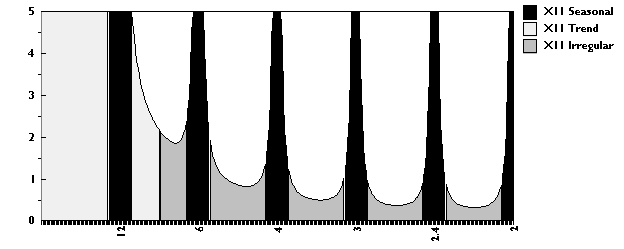Model Based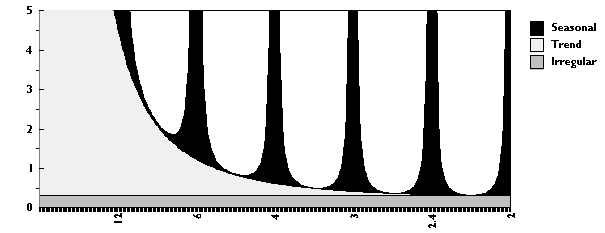Filter based methods assume that the each component exists only a certain cycle lengths. The longer cycles form the trend, the seasonal component is present at seasonal frequencies and the irregular component is defined as cycles of any other length.

Under a model based philosophy, the trend, seasonal and irregular component are present at all cycle lengths. The irregular component is of constant strength, the seasonal component peaks at seasonal frequencies and the trend component is strongest in the longer cycles.